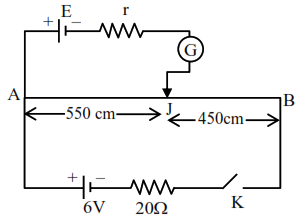# In the given figure,

Question:

In the given figure, there is a circuit of potentiometer of length $\mathrm{AB}=10 \mathrm{~m}$. The resistance per unit length is $0.1 \Omega$ per $\mathrm{cm}$. Across $\mathrm{AB}$, a battery of emf $E$ and internal resistance ' $r$ ' is connected. The maximum value of emf measured by this potentiometer is :1. $5 \mathrm{~V}$

2. $2.25 \mathrm{~V}$

3. $6 \mathrm{~V}$

4. $2.75 \mathrm{~V}$

Correct Option: 1

Solution:

Max. voltage that can be measured by this potentiometer will be equal to potential drop across $\mathrm{AB}$

$\mathrm{R}_{\mathrm{AB}}=10 \times 0.1 \times 100=100 \mathrm{ohm}$

$\therefore \mathrm{V}_{\mathrm{AB}}=\frac{6}{20+100} \times 100=6 \times \frac{100}{120}=5 \mathrm{~V}$